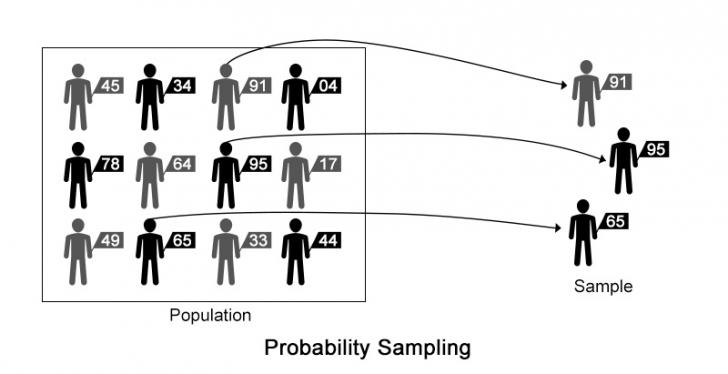When we choose certain items out of the whole population to analyze the data and draw a conclusion thereon, it is called sampling.

The way of sampling in which each item in the population has an equal chance (this chance is greater than zero) for getting selected is called probability sampling. Probability Sampling uses lesser reliance over the human judgment which makes the overall process free from over biasness.For instance, consider we need to sample 3 students from a group of 12. We firstly assign a random number to each of the element in the given data. After we assign the number, we mark the elements with the highest assigned random numbers in each row (56 in the first row, 92 in second and 63 in the third) Then we proceed for further analysis with the selected samples.

Since we assigned a number to each member of the population randomly, each one has an equal chance of being selected, making our process probabilistic.

• Cost Effective
As the task of assignment ogf random number to different items of population is over, the process is half done. This process saves cost and time to a great extent. We can take any number of samples from this process.

• Involves lesser degree of judgment
While assigning the number to an item of the population, the person assigns it in a random trend that makes the process of probability sampling more effective and more accurate.
• Comparatively easier way of sampling
Probability sampling does not involve any complex and long process. Thus this is an easier way for sampling.

• Less time consuming
This process is a simple and short process. This takes lesser time to complete. The saved time can be used for analysis and interpretation.

• Can be done even by non- technical persons
Assignment of Random number can be done by any kind of person after a short briefing as this does not involve any lengthy, complex and crucial process

• Sample representative of population
Probability Sampling uses random numbers which ensures that the samples vary as much as the population itself.